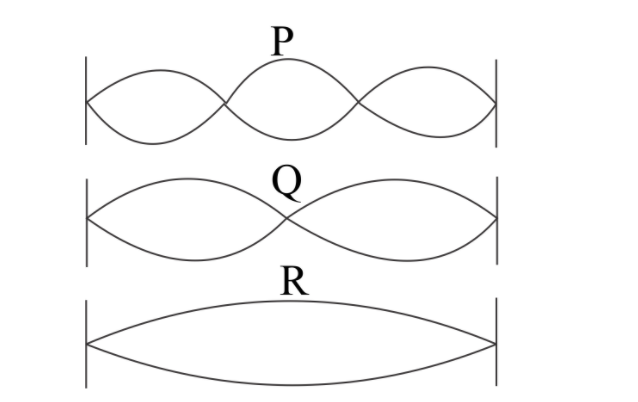Courses
Courses for Kids
Free study material
Free LIVE classes
More# The diagram below Shows three different modes of vibrations $P$, $Q$ and $R$ of the same string. The sound of which string will have maximum shrillness.Verified
290.1k+ views
Hint We know that the shrillness of a wave or vibration is directly proportional to frequency of wave. Loudness of vibration depends on its amplitude. Then the vibration with maximum frequency has maximum shrillness.

Complete step by step solution
We know that the shrillness of a wave or vibration is directly proportional to frequency of wave. Then the vibration with maximum frequency has maximum shrillness.
In the above diagram, vibration $P$ covers three loops, vibration $Q$ covers two loops when vibration $R$ covers only one loop.
Means vibration in string $P$ has more frequency than other two string $Q$ and $R$. Then frequency of vibration $P$ is maximum among all three vibrations.
We know maximum frequency means maximum shrillness. Then vibration $P$ has maximum shrillness.

Hence the answer is $P$.

Note: We know that sound is also the vibration of a particle. And loudness of sound depends on amplitude of sound wave and shrillness of sound depends on frequency of sound. To increase loudness of sound we just need to increase the amplitude of sound. And we never change the frequency of sound because all information is just a varying frequency of sound wave. If we do so the message in sound changes.
Last updated date: 03rd Jun 2023
Total views: 290.1k
Views today: 5.46k